Tip: Other languages are Google-Translated. You can visit the English version of this link.orRegisteror

## How to quickly sum every other or nth row/column in Excel?

As we all know, we can apply the Sum function to add a list of cells, but sometimes we need to sum every other cell for some purpose, and Excel has no standard function allow us to sum every nth cell. In this situation, how could we sum every other or nth row / column in Excel?

Sum every other or nth row/column with Formulas

Sum every other or nth row/column with User Defined Function

 Select every other or nth row / column and then get the calculations as you need: To sum, average or count every other or nth row or column, Kutools for Excel's Select Interval Rows & Columns feature may help you to select every other or nth row firstly, and then get the calculations at the bottom of the status bar.       Read more...          Free Dowload.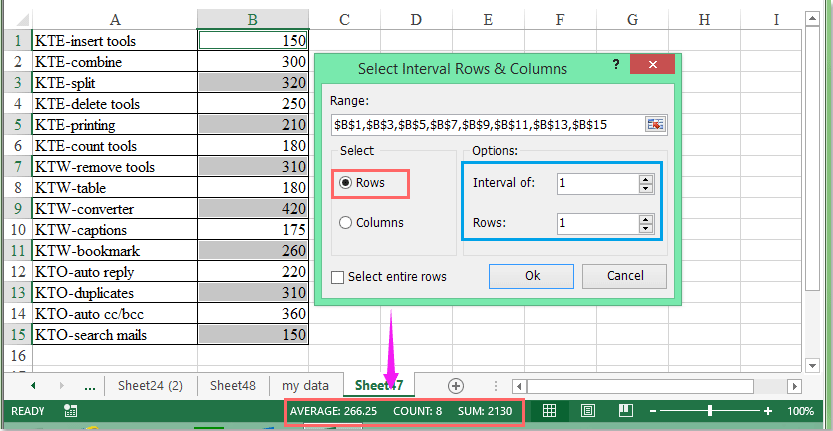Kutools for Excel: with more than 200 handy Excel add-ins, free to try with no limitation in 60 days.###### Save 50% of your time, and reduce thousands of mouse clicks for you every day!

Through the following example, I will show you how to apply the formulas that sum every other cell.

1. Use the array formulas to sum every other row or column

In a blank cell, please enter this formula: =SUM(IF(MOD(ROW(\$B\$1:\$B\$15),2)=0,\$B\$1:\$B\$15,0)), then press Ctrl + Shift + Enter keys, and every other cell values in the column B have been summed. See screenshots: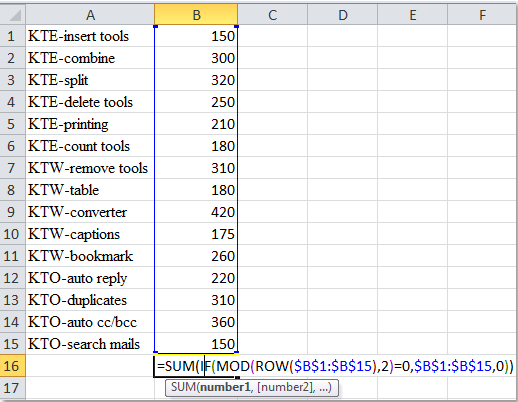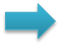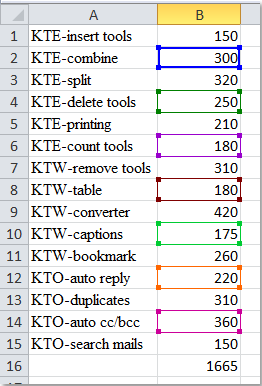Notes:

1. In the above array formula, you can change the number 2 to 3, 4, 5…, it means sum every 3rd row, every 4th row, every 5th row…

2. If you want to sum every other column, you can input the following formula: =SUM(IF(MOD(COLUMN(\$A\$1:\$O\$1),2)=0,\$A\$1:\$O\$1,0)), and press Ctrl + Shift + Enter keys to get the result. See screenshot: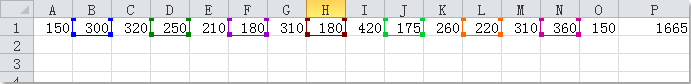2. Use the formulas to sum every other row or column

Here is another formula can help you to sum every other or nth cell in a worksheet.

In an empty cell, please enter this formula: =SUMPRODUCT((MOD(ROW(\$B\$1:\$B\$15),3)=0)*(\$B\$1:\$B\$15)). And then press Enter key, and every 3rd cell has been added. See screenshots: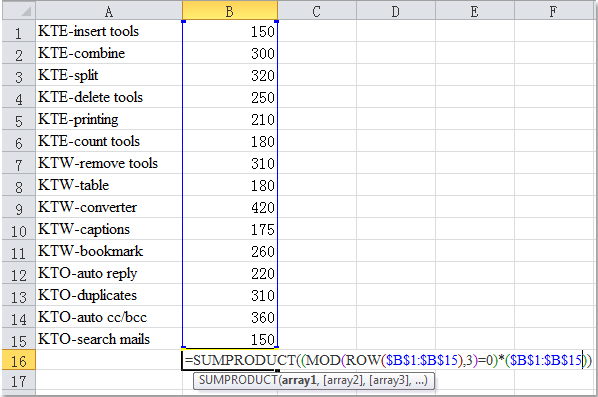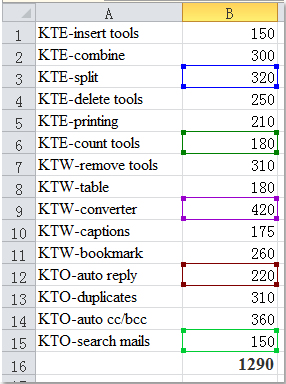Notes:

1. In the above formula, you can change the number 3 to 4, 5, 6…, it means sum every 4th row, every 5th row, every 6th row…

2. If you want to sum every other column, you can input the following formula: =SUMPRODUCT((MOD(COLUMN(\$A\$1:\$O\$1),3)=0)*(\$A\$1:\$O\$1)).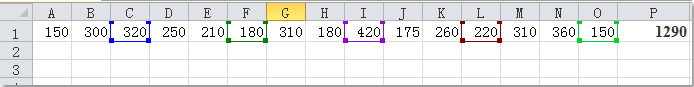If you want to know more details about these formulas, please go to visit the following websites:

http://www.xl-central.com/sum-every-2nd-row.html

http://www.excel-easy.com/examples/sum-every-nth-row.html

The following user defined function also can help you to add every other or nth cell in Excel.

1. Hold down the ALT + F11 keys, and it opens the Microsoft Visual Basic for Applications window.

2. Click Insert > Module, and paste the following code in the Module window.

```Function SumIntervalRows(WorkRng As Range, interval As Integer) As Double
'Update 20130907
Dim arr As Variant
Dim total As Double
total = 0
arr = WorkRng.Value
For i = interval To UBound(arr, 1) Step interval
total = total + arr(i, 1)
Next
SumIntervalRows = total
End Function
Function SumIntervalCols(WorkRng As Range, interval As Integer) As Double
Dim arr As Variant
Dim total As Double
total = 0
arr = WorkRng.Value
For j = interval To UBound(arr, 2) Step interval
total = total + arr(1, j)
Next
SumIntervalCols = total
End Function
```

3. Then save this code, and type the following formula into a blank cell as you need, see screenshots:

For summing every fourth row: =SumIntervalRows (B1:B15,4)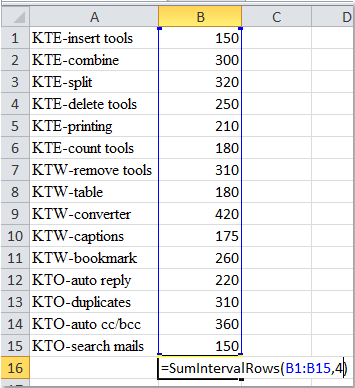For summing every fourth column: =SumIntervalCols (A1:O1,4)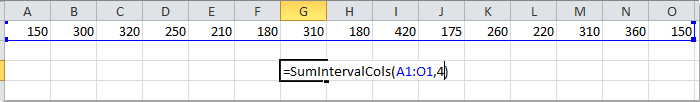4. Then press Enter key, you will get the calculation. See screenshots:

Sum every fourth row: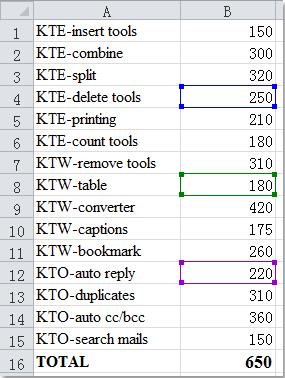Sum every fourth column: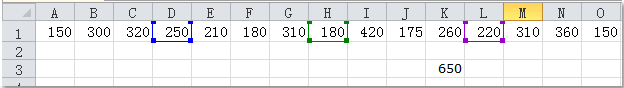Note: You can change the number 4 to any other numbers, such as 2, 3, 5… it means sum every second row, every third row, every fifth row or column.

Maybe both the formulas and user defined function are somewhat difficult for most of you, here I can introduce you an easier way to solve this task.

With Kutools for Excel’s Select Interval Rows & Columns, you can select every other or nth cell that you need first, and then give the selected cells a defined name, at last, using the Sum function to sum the cells.

 : with more than 300 handy Excel add-ins, free to try with no limitation in 60 days.

After installing Kutools for Excel, please do as the following steps:

1. Select the column that you want to sum every other or nth cell.

2. Click Kutools > Select > Select Interval Rows & Columns, see screenshot: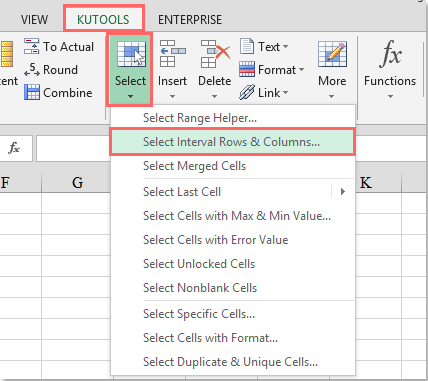3. In the Select Interval Rows & Columns dialog box, specify the operations as you need, see screenshot: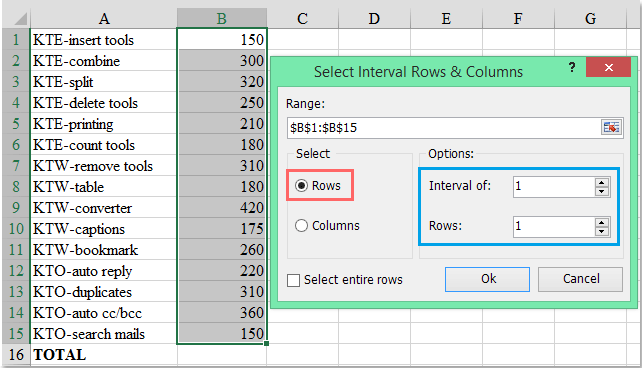4. Click OK, and every other row has been selected from the first row, and now, you can view the calculated results, such as average, sum, count are displayed at the bottom of the status bar. See screenshot: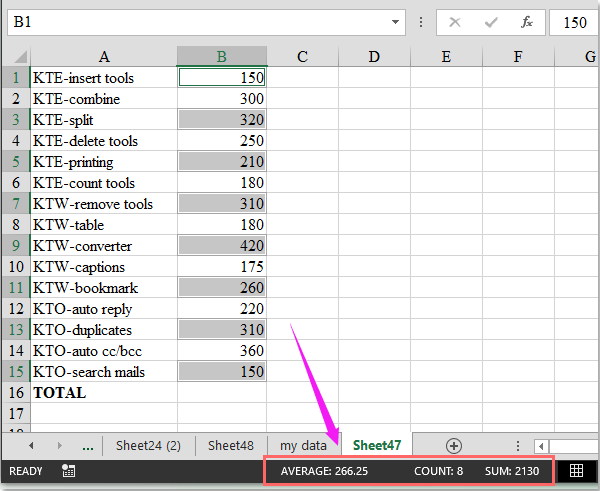Notes:

1. With this function, you can set the number 2, 3, 4… in Interval of option, and you will select the cells with the interval of 2, 3, 4 rows starting from the first row of the range in the step 3.

2. With the above steps, you can also sum every other or nth column as you need.

Kutools for Excel: with more than 200 handy Excel add-ins, free to try with no limitation in 60 days.

Related articles:

How to sum only filtered or visible cells in Excel?

How to sum the absolute values in Excel?

### Kutools for Excel Solves Most of Your Problems, and Increases Your Productivity by 80%

• Reuse: Quickly insert complex formulas, charts and anything that you have used before; Encrypt Cells with password; Create Mailing List and send emails...
• Super Formula Bar (easily edit multiple lines of text and formula); Reading Layout (easily read and edit large numbers of cells); Paste to Filtered Range...
• Merge Cells/Rows/Columns without losing Data; Split Cells Content; Combine Duplicate Rows/Columns... Prevent Duplicate Cells; Compare Ranges...
• Select Duplicate or Unique Rows; Select Blank Rows (all cells are empty); Super Find and Fuzzy Find in Many Workbooks; Random Select...
• Exact Copy Multiple Cells without changing formula reference; Auto Create References to Multiple Sheets; Insert Bullets, Check Boxes and more...
• Extract Text, Add Text, Remove by Position, Remove Space; Create and Print Paging Subtotals; Convert Between Cells Content and Comments...
• Super Filter (save and apply filter schemes to other sheets); Advanced Sort by month/week/day, frequency and more; Special Filter by bold, italic...
• Combine Workbooks and WorkSheets; Merge Tables based on key columns; Split Data into Multiple Sheets; Batch Convert xls, xlsx and PDF...
• More than 300 powerful features. Supports Office/Excel 2007-2019 and 365. Supports all languages. Easy deploying in your enterprise or organization. Full features 30-day free trial.### Office Tab Brings Tabbed interface to Office, and Make Your Work Much Easier

• Enable tabbed editing and reading in Word, Excel, PowerPoint, Publisher, Access, Visio and Project.
• Open and create multiple documents in new tabs of the same window, rather than in new windows.
• Increases your productivity by 50%, and reduces hundreds of mouse clicks for you every day!Say something here...
symbols left.
###### or post as a guest, but your post won't be published automatically.
• To post as a guest, your comment is unpublished.
· 2 months ago
These vma tutorials are the best thing ever. Very helpful thank you guys!
• To post as a guest, your comment is unpublished.
· 7 months ago
• To post as a guest, your comment is unpublished.
· 6 months ago
Hi, Nilesh,
If you want to sum the cell values in all odd row, the below formula may help you! (Please change the cell references to your need.)

=SUMPRODUCT(B1:B15,MOD(ROW(B1:B15)+0,2))

• To post as a guest, your comment is unpublished.
· 2 years ago
Is there a way to add another criteria to this formula? I want to add every 9th row that is great than -40. I would appreciate your help. Thanks in advance!
• To post as a guest, your comment is unpublished.
· 3 years ago
Used the visual basic programming – excellent!

The other options worked fine except for row n.º 1.
• To post as a guest, your comment is unpublished.
· 3 years ago
sir
I have used visual basic for SumIntervalCols function as stated above and saved the file as macro enable worksheet.
when next time open the file the user define command is missing from drop down function menu

regards
D KUMAR
• To post as a guest, your comment is unpublished.
· 3 years ago
This method does not work for me. I entered the 15 numbers in the column B (B1:B15) as in the example and copy the formula to a blank cell, the result is 0. If I change the if statement to IF(MOD(ROW(\$B\$1:\$B\$15),2)=1, the result is 3795 that is sum(B1:B15). What is wrong?
• To post as a guest, your comment is unpublished.
· 4 years ago
This formula is easily modified to work with either ranges starting with odd or even numbered rows/columns. For example: =SUM(IF(MOD(ROW(\$B\$1:\$B\$15),2)=0,\$B\$1:\$B\$15,0)) will sum only even numbered rows in the specified range, but =SUM(IF(MOD(ROW(\$B\$1:\$B\$15),2)=1,\$B\$1:\$B\$15,0)) will sum only odd numbered row. This is because of the way the MOD function works. MOD(4,2) returns 0 because 2 divides into 4 with no remainders because 4 is an even number, but MOD(5,2) returns 1 because 2 into 5 leaves 1 remained.
• To post as a guest, your comment is unpublished.
· 4 years ago
i am very interested now more about excel so please guide me.
so, in will adding in last u use 4 why.
• To post as a guest, your comment is unpublished.
· 4 years ago
sum of columns works as well, but you need to start from column A otherwise it returns a wrong result.
• To post as a guest, your comment is unpublished.
· 4 years ago
HOPE THIS HELP SORT OUT THE ISSUE
• To post as a guest, your comment is unpublished.
· 5 years ago
This was very helpful. I was looking form something that would total Ro1 1 col 1, 4, 7, etc. Using the SumIntervalCols didn't work correctly so by just changing the For loop to: For j = 1 To UBound(arr, 2) Step interval gave me what I wanted, e.g. (F2:BH2,3). Now I wanted to average the totals in every third cell so I added the function:

Function CountIntervalCols(WorkRng As Range, interval As Integer) As Double
Dim arr As Variant
Dim total As Double
total = 0
arr = WorkRng.Value
For j = 1 To UBound(arr, 2) Step interval
If (Not IsEmpty(arr(1, j)) And IsNumeric(arr(1, j))) Then
If arr(1, j) > 0 Then
total = total + 1
End If
End If
Next
CountIntervalCols = total
End Function

Then it was simple to create an AvgIntervalCols:

Function AvgIntervalCols(WorkRng As Range, interval As Integer) As Double
Dim totalsum As Double
Dim totalcount As Double
totalsum = SumIntervalCols(WorkRng, interval)
totalcount = CountIntervalCols(WorkRng, interval)
If totalcount = 0 Then
totalsum = 0
Else
totalsum = totalsum / totalcount
End If
AvgIntervalCols = totalsum
End Function

Thanks!
• To post as a guest, your comment is unpublished.
· 5 years ago
This is a wonderful article! I didn't know that the MOD function can handle an array argument. I enjoyed the formula solution very much, it is simply brilliant!
• To post as a guest, your comment is unpublished.
· 5 years ago
Hi,

this is helpful, but the formula is bonkers (at least if you try to apply it to columns) Column(REGION) returns the column index of the 1st column in the region. So the formula totally does not work for columns...

But it is helpful to start you working in the right direction.
• To post as a guest, your comment is unpublished.
· 5 years ago
Hi
I tried it - but this formula
=SUM(IF(MOD(ROW(\$B\$1:\$B\$15),2)=0,\$B\$1:\$B\$15))
functions only IF you start at an uneven row.Otherwise it gives a wrong result. Try it,insert a row before row one.
ADVICE: instead insert the 2nd or 3rd row to sum (it is a variable, insert a reference Cell (B20) or so and insert there which every row you like to sum.
Anyway, I wonder for what the heck you use such a formula???
best regards
kraems